# matplotlib.pyplot.colorbar¶

`matplotlib.pyplot.``colorbar`(mappable=None, cax=None, ax=None, **kw)[source]

Add a colorbar to a plot.

Function signatures for the `pyplot` interface; all but the first are also method signatures for the `colorbar()` method:

```colorbar(**kwargs)
colorbar(mappable, **kwargs)
colorbar(mappable, cax=cax, **kwargs)
colorbar(mappable, ax=ax, **kwargs)
```
Parameters: mappable : The `Image`, `ContourSet`, etc. to which the colorbar applies; this argument is mandatory for the Figure `colorbar()` method but optional for the pyplot `colorbar()` function, which sets the default to the current image. cax : `Axes` object, optional Axis into which the colorbar will be drawn ax : `Axes`, list of Axes, optional Parent axes from which space for a new colorbar axes will be stolen. If a list of axes is given they will all be resized to make room for the colorbar axes. use_gridspec : bool, optional If cax is `None`, a new cax is created as an instance of Axes. If ax is an instance of Subplot and use_gridspec is `True`, cax is created as an instance of Subplot using the grid_spec module. :class:`~matplotlib.colorbar.Colorbar` instance See also its base class, `ColorbarBase`. Call the `set_label()` method to label the colorbar.

Notes

Additional keyword arguments are of two kinds:

axes properties:

Property Description
orientation vertical or horizontal
fraction 0.15; fraction of original axes to use for colorbar
pad 0.05 if vertical, 0.15 if horizontal; fraction of original axes between colorbar and new image axes
shrink 1.0; fraction by which to multiply the size of the colorbar
aspect 20; ratio of long to short dimensions
anchor (0.0, 0.5) if vertical; (0.5, 1.0) if horizontal; the anchor point of the colorbar axes
panchor (1.0, 0.5) if vertical; (0.5, 0.0) if horizontal; the anchor point of the colorbar parent axes. If False, the parent axes’ anchor will be unchanged

colorbar properties:

Property Description
extend [ ‘neither’ | ‘both’ | ‘min’ | ‘max’ ] If not ‘neither’, make pointed end(s) for out-of- range values. These are set for a given colormap using the colormap set_under and set_over methods.
extendfrac [ None | ‘auto’ | length | lengths ] If set to None, both the minimum and maximum triangular colorbar extensions with have a length of 5% of the interior colorbar length (this is the default setting). If set to ‘auto’, makes the triangular colorbar extensions the same lengths as the interior boxes (when spacing is set to ‘uniform’) or the same lengths as the respective adjacent interior boxes (when spacing is set to ‘proportional’). If a scalar, indicates the length of both the minimum and maximum triangular colorbar extensions as a fraction of the interior colorbar length. A two-element sequence of fractions may also be given, indicating the lengths of the minimum and maximum colorbar extensions respectively as a fraction of the interior colorbar length.
extendrect bool If False the minimum and maximum colorbar extensions will be triangular (the default). If True the extensions will be rectangular.
spacing [ ‘uniform’ | ‘proportional’ ] Uniform spacing gives each discrete color the same space; proportional makes the space proportional to the data interval.
ticks [ None | list of ticks | Locator object ] If None, ticks are determined automatically from the input.
format [ None | format string | Formatter object ] If None, the `ScalarFormatter` is used. If a format string is given, e.g., ‘%.3f’, that is used. An alternative `Formatter` object may be given instead.
drawedges bool Whether to draw lines at color boundaries.

The following will probably be useful only in the context of indexed colors (that is, when the mappable has norm=NoNorm()), or other unusual circumstances.

Property Description
boundaries None or a sequence
values None or a sequence which must be of length 1 less than the sequence of boundaries. For each region delimited by adjacent entries in boundaries, the color mapped to the corresponding value in values will be used.

If mappable is a `ContourSet`, its extend kwarg is included automatically.

The shrink kwarg provides a simple way to scale the colorbar with respect to the axes. Note that if cax is specified it determines the size of the colorbar and shrink and aspect kwargs are ignored.

For more precise control, you can manually specify the positions of the axes objects in which the mappable and the colorbar are drawn. In this case, do not use any of the axes properties kwargs.

It is known that some vector graphics viewer (svg and pdf) renders white gaps between segments of the colorbar. This is due to bugs in the viewers not matplotlib. As a workaround the colorbar can be rendered with overlapping segments:

```cbar = colorbar()
cbar.solids.set_edgecolor("face")
draw()
```

However this has negative consequences in other circumstances. Particularly with semi transparent images (alpha < 1) and colorbar extensions and is not enabled by default see (issue #1188).

## Examples using `matplotlib.pyplot.colorbar`¶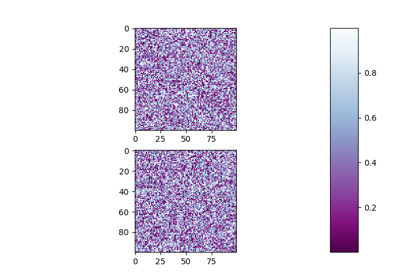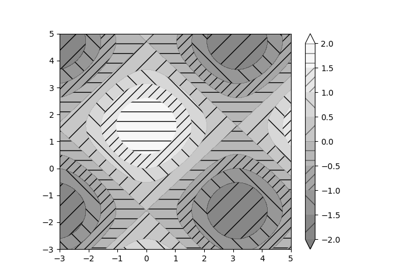Contourf Hatching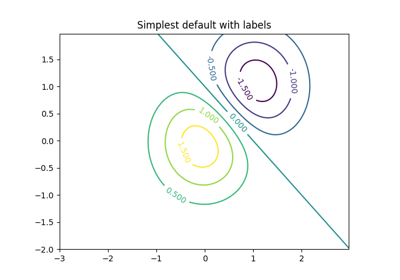Contour Demo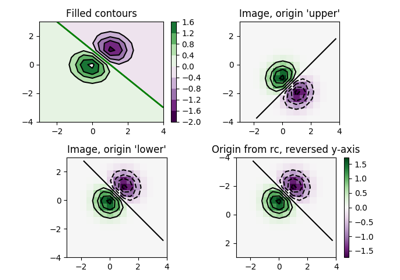Contour Image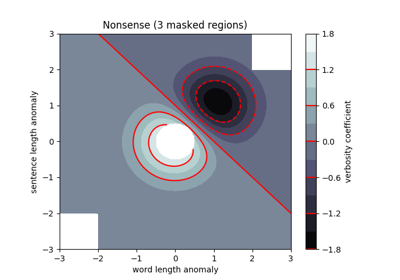Contourf Demo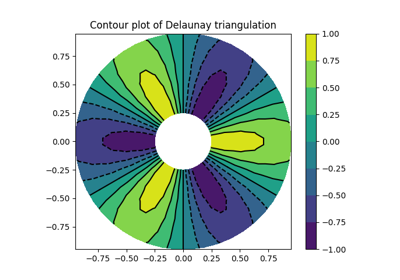Tricontour Demo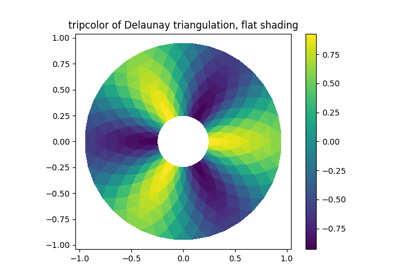Tripcolor Demo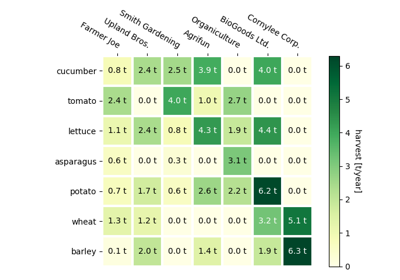Creating annotated heatmaps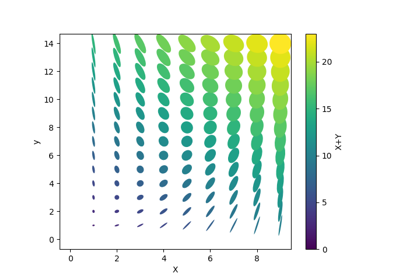Ellipse Collection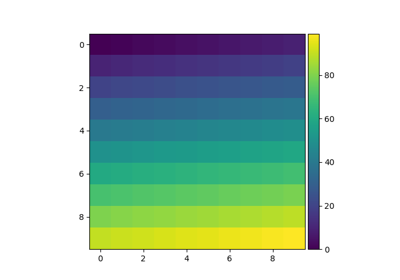Simple Colorbar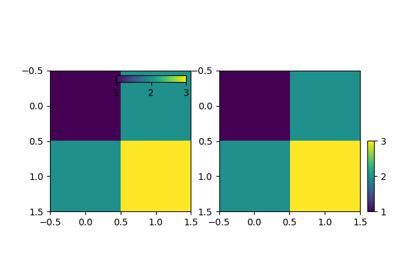Demo Colorbar With Inset Locator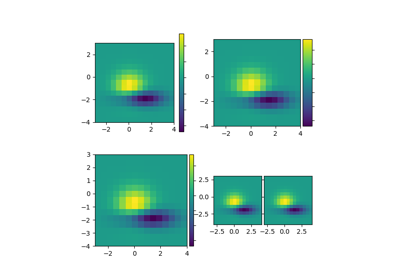Demo Axes Divider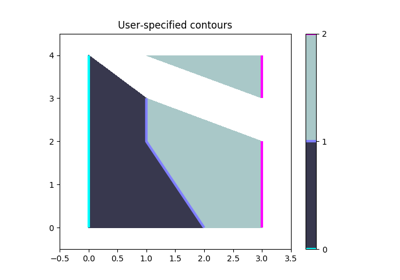Manual Contour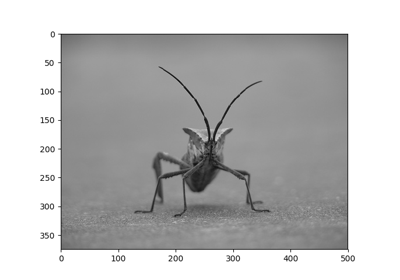Image tutorial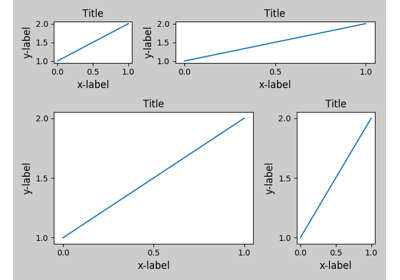Tight Layout guide